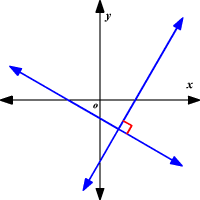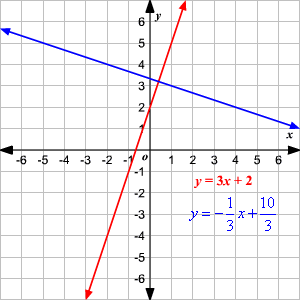# Perpendicular Lines and Slopes

Perpendicular lines are lines that intersect at right angles.

If you multiply the slopes of two perpendicular lines in the plane, you get $-1$ . That is, the slopes of perpendicular lines are opposite reciprocals .(Exception: Horizontal and vertical lines are perpendicular, though you can't multiply their slopes, since the slope of a vertical line is undefined.)

We can write the equation of a line perpendicular to a given line if we know a point on the line and the equation of the given line.

Example :

Write the equation of a line that passes through the point $\left(1,3\right)$ and is perpendicular to the line $y=3x+2$ .

Perpendicular lines are lines that intersect at right angles.

The slope of the line with equation $y=3x+2$ is $3$ . If you multiply the slopes of two perpendicular lines, you get $-1$ .

$3\cdot \left(-\frac{1}{3}\right)=-1$

So, the line perpendicular to $y=3x+2$ has the slope $-\frac{1}{3}$ .

Now use the point-slope form to find the equation.

$y-{y}_{1}=m\left(x-{x}_{1}\right)$

We have to find the equation of the line which has the slope $-\frac{1}{3}$ and passes through the point $\left(1,3\right)$ . So, replace $m$ with $-\frac{1}{3}$ , ${x}_{1}$ with $1$ , and ${y}_{1}$ with $3$ .

$y-3=-\frac{1}{3}\left(x-1\right)$

Use the distributive property .

$y-3=-\frac{1}{3}x+\frac{1}{3}$

Add $3$ to each side.

$\begin{array}{l}y-3+3=-\frac{1}{3}x+\frac{1}{3}+3\\ \text{\hspace{0.17em}}\text{\hspace{0.17em}}\text{\hspace{0.17em}}\text{\hspace{0.17em}}\text{\hspace{0.17em}}\text{\hspace{0.17em}}\text{\hspace{0.17em}}\text{\hspace{0.17em}}\text{\hspace{0.17em}}\text{\hspace{0.17em}}\text{\hspace{0.17em}}\text{\hspace{0.17em}}y=-\frac{1}{3}x+\frac{10}{3}\end{array}$

Therefore, the line $y=-\frac{1}{3}x+\frac{10}{3}$ is perpendicular to the line $y=3x+2$ and passes through the point $\left(1,3\right)$ .